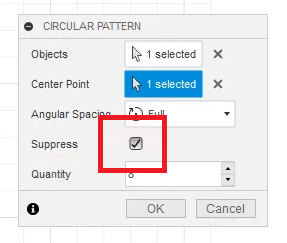# Fusion 360 2D Sketching

Fundamentals at 2D level in Fusion 360 using basic shaped., we would use the `X` (red) and `Y` (green) plains and the 3D printer will extrude into `Z` (blue). `Create` - > `Create Scetch` and the select the plain between red and green.

We need to be deliberate about position and dimension so that the sketch always has the little red lock indicating its `fully constrained` and the object we added goes black (and not blue).

## Circle (at origin)

`CREATE` - > `Circle` -> `Center Diameter Circle` -> select position (origin) -> provide dimension.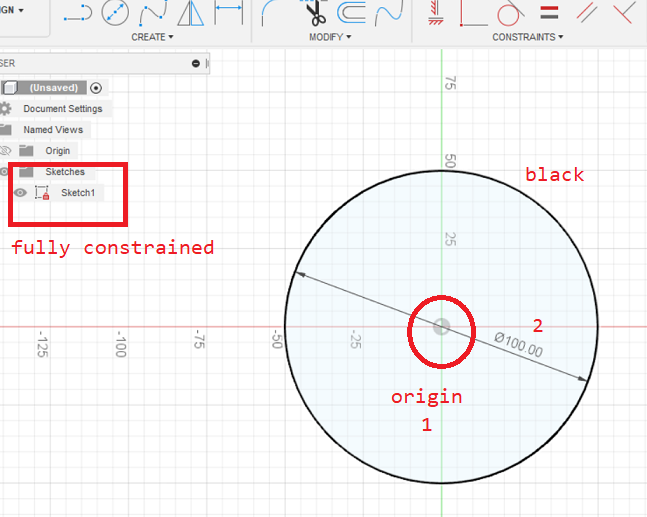## Circle (on construction lines)

`CREATE` - > `Line` -> set line type `construction` -> select position (origin) -> align to plain (red or green) -> provide dimension.

`CREATE` - > `Circle` -> select construction line position -> provide dimension.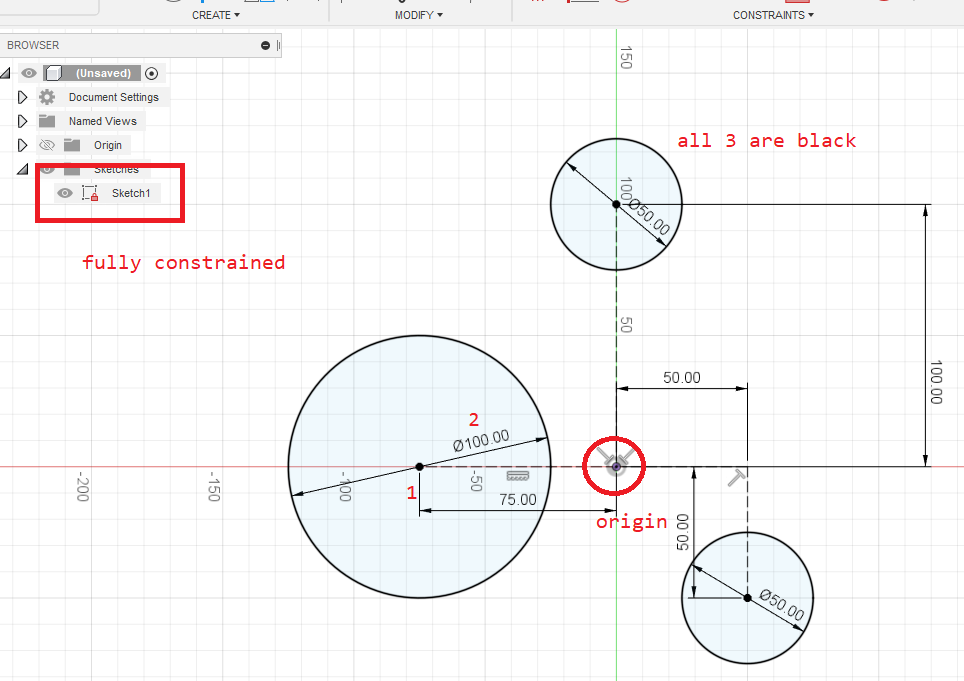## Rectangle

`CREATE` - > `Line` -> set line type `construction` -> select position (origin) -> align to plain (red or green) -> provide dimension.

`CREATE` - > `Rectangle` -> `2 Point Rectangle` -> select construction line position -> provide dimension -> tab -> provide 2nd dimension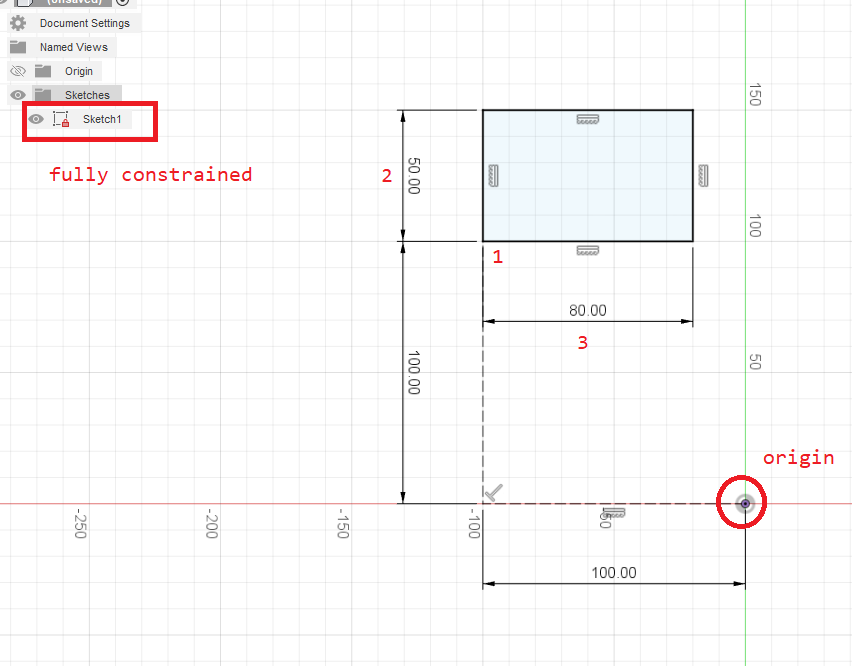## Arc (from origin along construction line)

Create 2 constructions lines along red, 100mm and 50mm

`CREATE` - > `Arc` -> `3 Point Arc` -> select origin -> select the end of the 100mm construction line

Create constraint of type `coincident`

`CONSTRAINT` -> `Coincident` -> select the end of the 50mm construction line -> select the center point of the arc## Polygon

Create construction lines simliar to the above

`CREATE` - > `Polygon` -> `Circumscribed Polygon` -> select the construction line point -> drag and set the radius -> tab and set the amount of sides. Repeat and create another 2 polygons to the right and below

Create constraint of type `Horizontal/Vertial` (for the first polygon)

Select the polygons top line -> select `CONSTRAINT` -> `Horizontal/Vertial`

Create constraint of type `Colinear`

`CONSTRAINT` -> `Colinear` -> select point in the middle of the polygon -> select a corner of the polygon -> select point of construction line outside of the polygon

Create constraint of type `Parallel`

`CONSTRAINT` -> `Parallel` -> select a line from the polygon -> select a construction line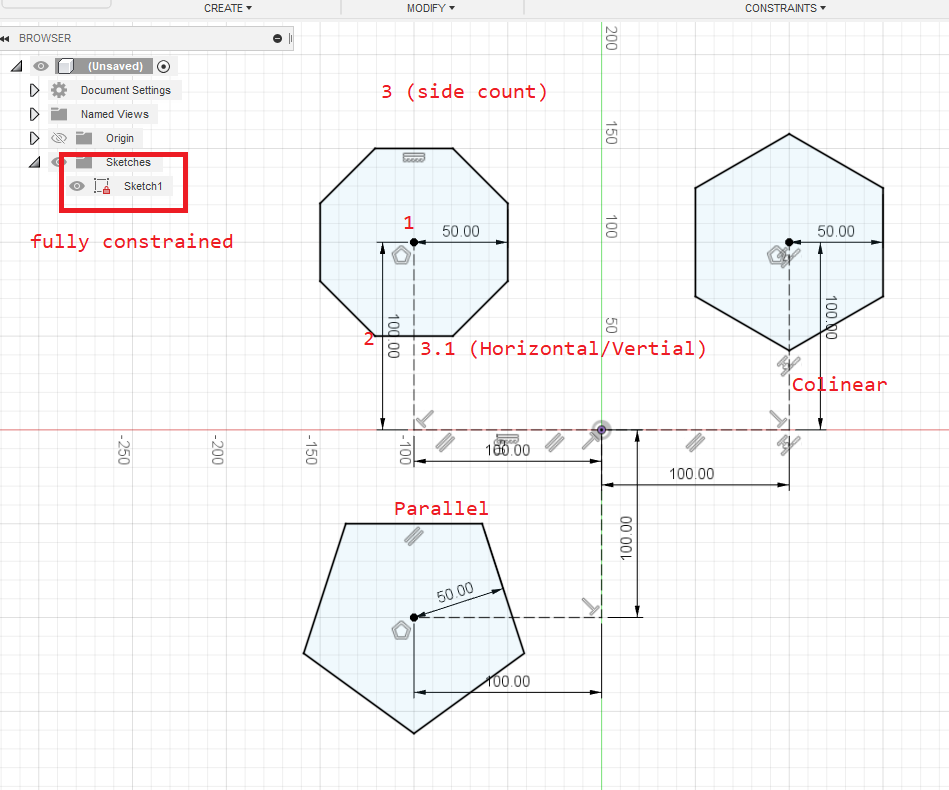Circumscribed (outside the circle) | Inscribed (inside the circle)

## Ellipse

`CREATE` - > `Ellipse` -> select the construction line point `` -> release and select the tip of the at the next construction line `` -> set the dimension ``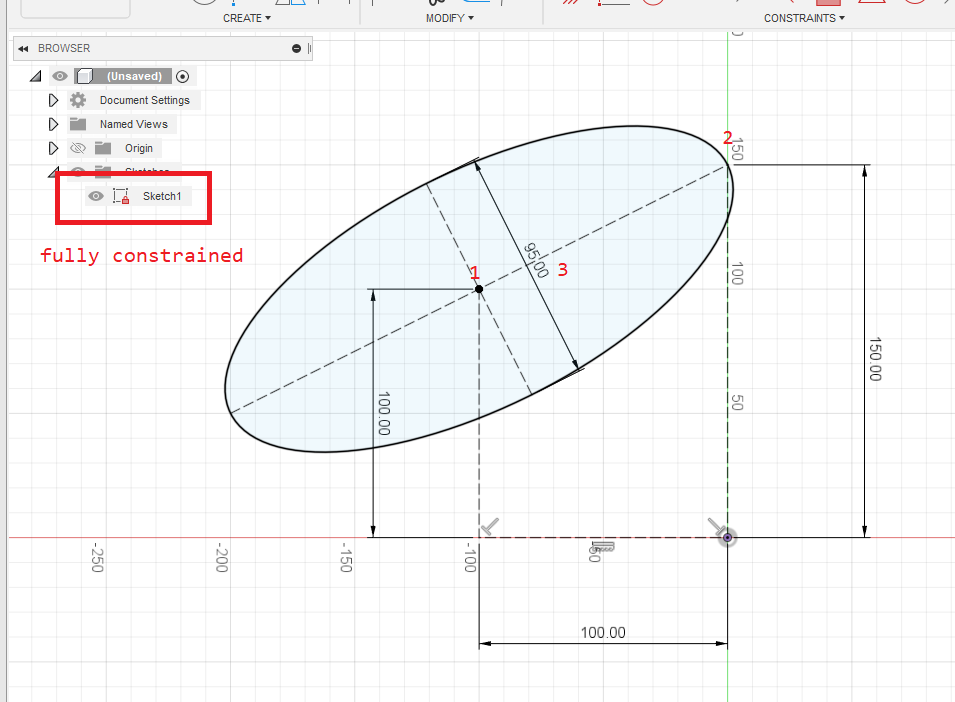## Slot

Requires a square with 2 construction lines deviding it into 4 equal parts, then from the center 2 additional construction lines (these are the `25mm` ones)

`CREATE` - > `Slot` -> `Center to Center Slot` -> select construction line point `` -> release and select construction line point `` -> then set the dimension (this is the `25mm` radius)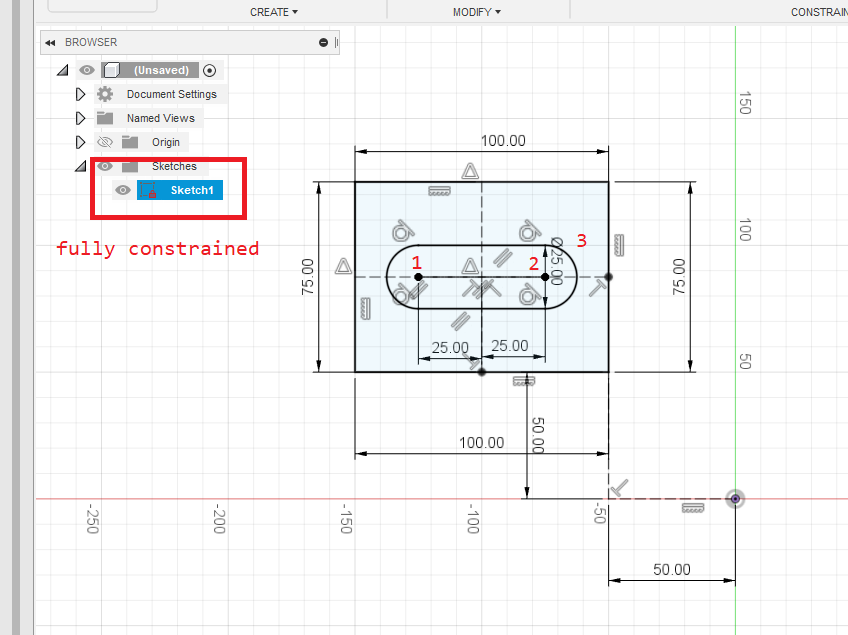## Conic Curve

Requires the 3 construction lines shown as `100.00`, `100.00` and `50.00`

`CREATE` - > `Conic Curve` -> select construction line at point `` -> construction line at point `` -> then construction line at point `` -> then set the dimension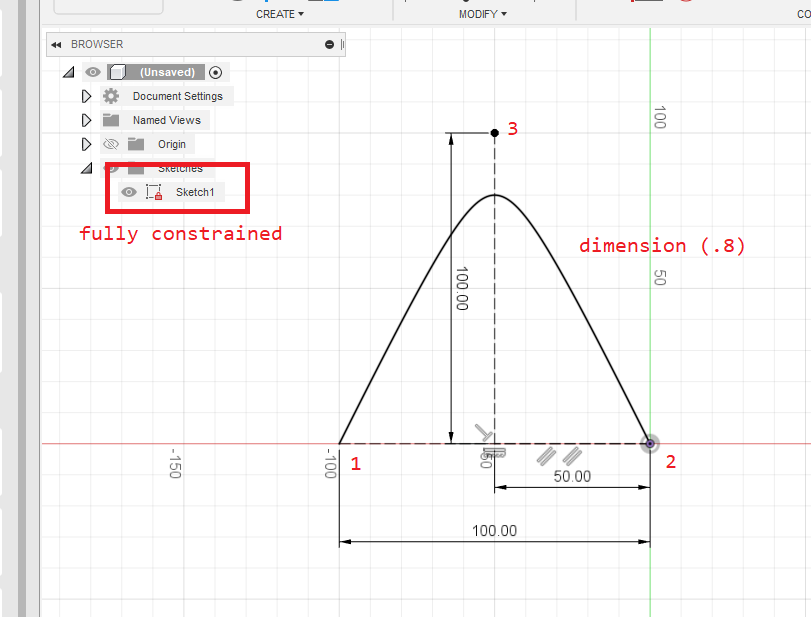## Mirror

This mirrors the selection and applys any new changes automagically. Here I mirrored the conic curve above.

`CREATE` - > `Mirrow` -> select the object (can be any object) at `` -> select the line at `` -> the mirror then appears on the right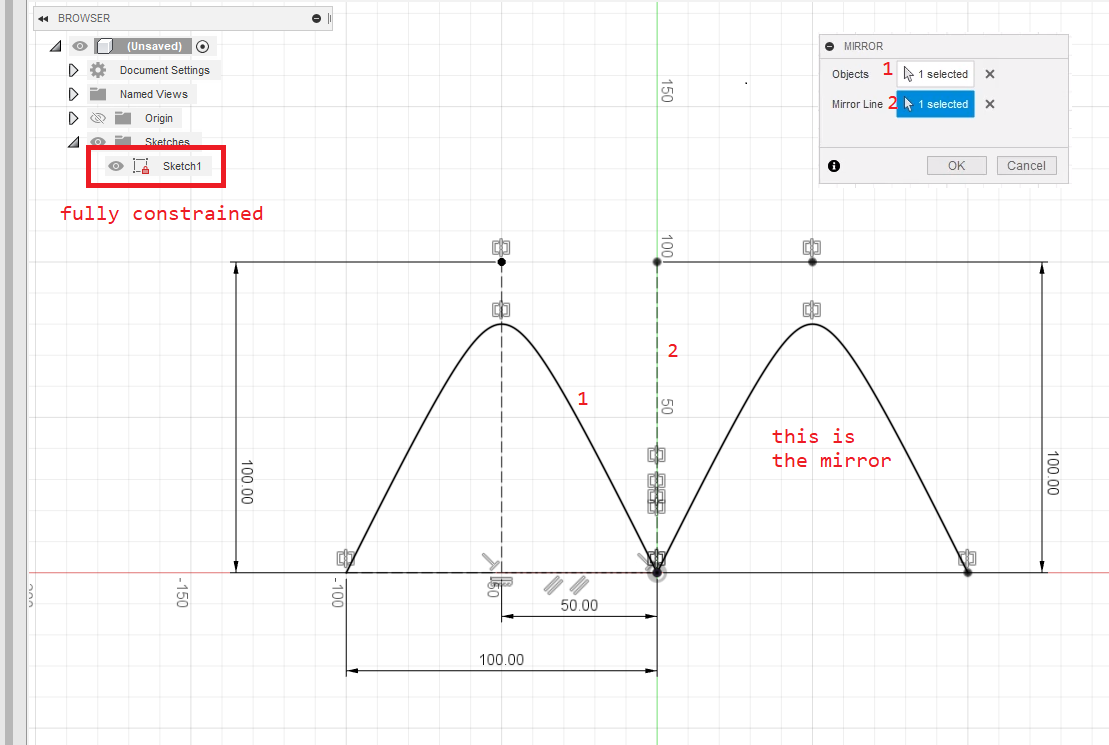## Circular Pattern

This requires 1 construction line and then the object to create in a circular pattern.

`CREATE` - > `Circular Pattern` -> select the object at `` -> select the center point at `` -> set the quantity and click okCan also create pattern with missing elements by selecting suppress and then unchecking elements